# Add Only Distinct Positive Values with VBA

In this article, you will learn how to add only distinct positive values in excel using VBA code.

Question): I want a macro to sum all of the distinct positive numbers in a specified range which includes negative & positive numbers.

Following is the snapshot of the data we have: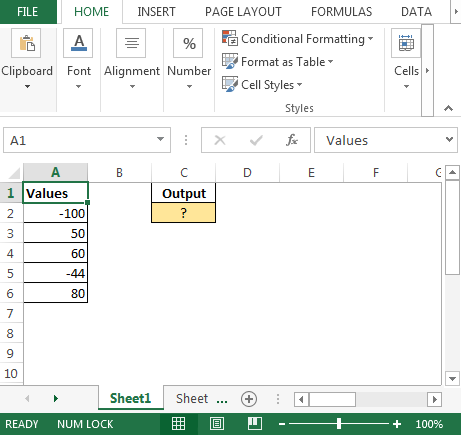We need to follow the below steps:

• Click on Developer tab
• From Code group select Visual Basic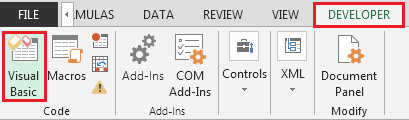Enter the following code in the standard module:-

Function AddUnique(ByRef inputrange As Range, _

Optional IgnoreText As Boolean = True, _

Optional IgnoreError As Boolean = True, _

Optional IgnoreNegativenumbers As Boolean = True)

Dim distinctnumbers As Double

Dim cell As Range

Dim dict As Object

Set dict = CreateObject("Scripting.dictionary")

distinctnumbers = 0

For Each cell In inputrange.Resize(inputrange.Rows.Count, 1)

cval = cell.Value

If IgnoreText Then

If Not (VBA.IsNumeric(cval)) Then cval = 0

Else

Exit Function

End If

If IgnoreError Then

If IsError(cval) Then cval = 0

Else

Exit Function

End If

If IgnoreNegativenumbers Then

If cval < 0 Then cval = 0

Else

Exit Function

End If

If Not dict.Exists(cval) Then

distinctnumbers = distinctnumbers + cval

End If

Next

End Function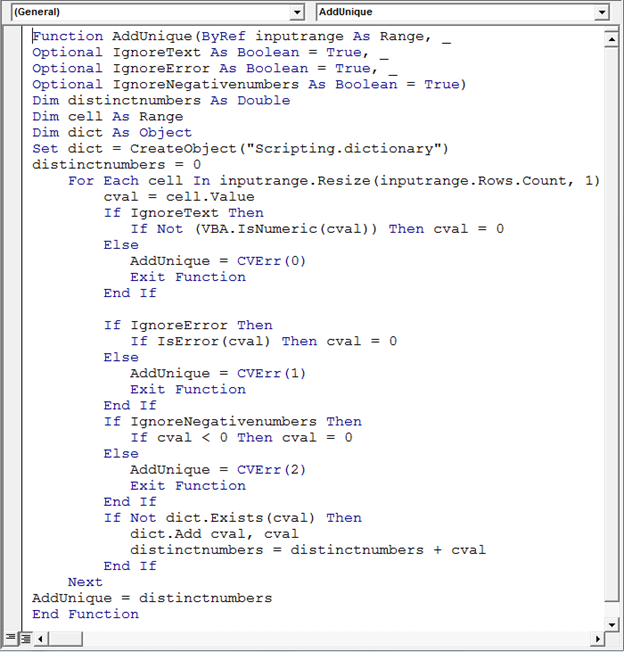• In cell C2 the formula would be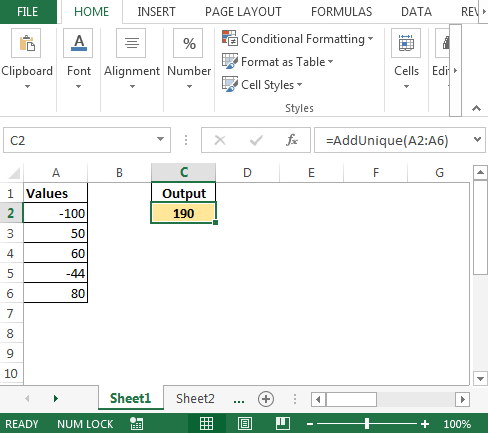• To check whether code ignores repeated value or not; let us change the value in cell A1 to 50 & see the result.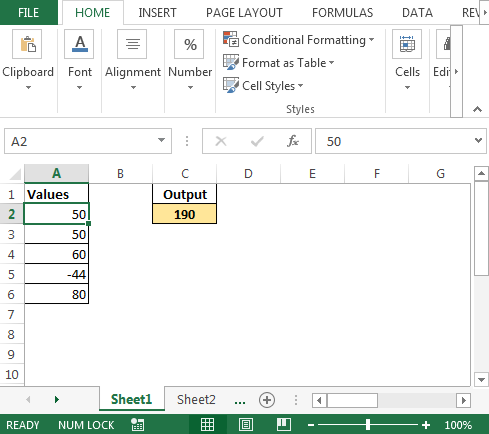In this way we can add distinct positive values through macro code.

Terms and Conditions of use

The applications/code on this site are distributed as is and without warranties or liability. In no event shall the owner of the copyrights, or the authors of the applications/code be liable for any loss of profit, any problems or any damage resulting from the use or evaluation of the applications/code.